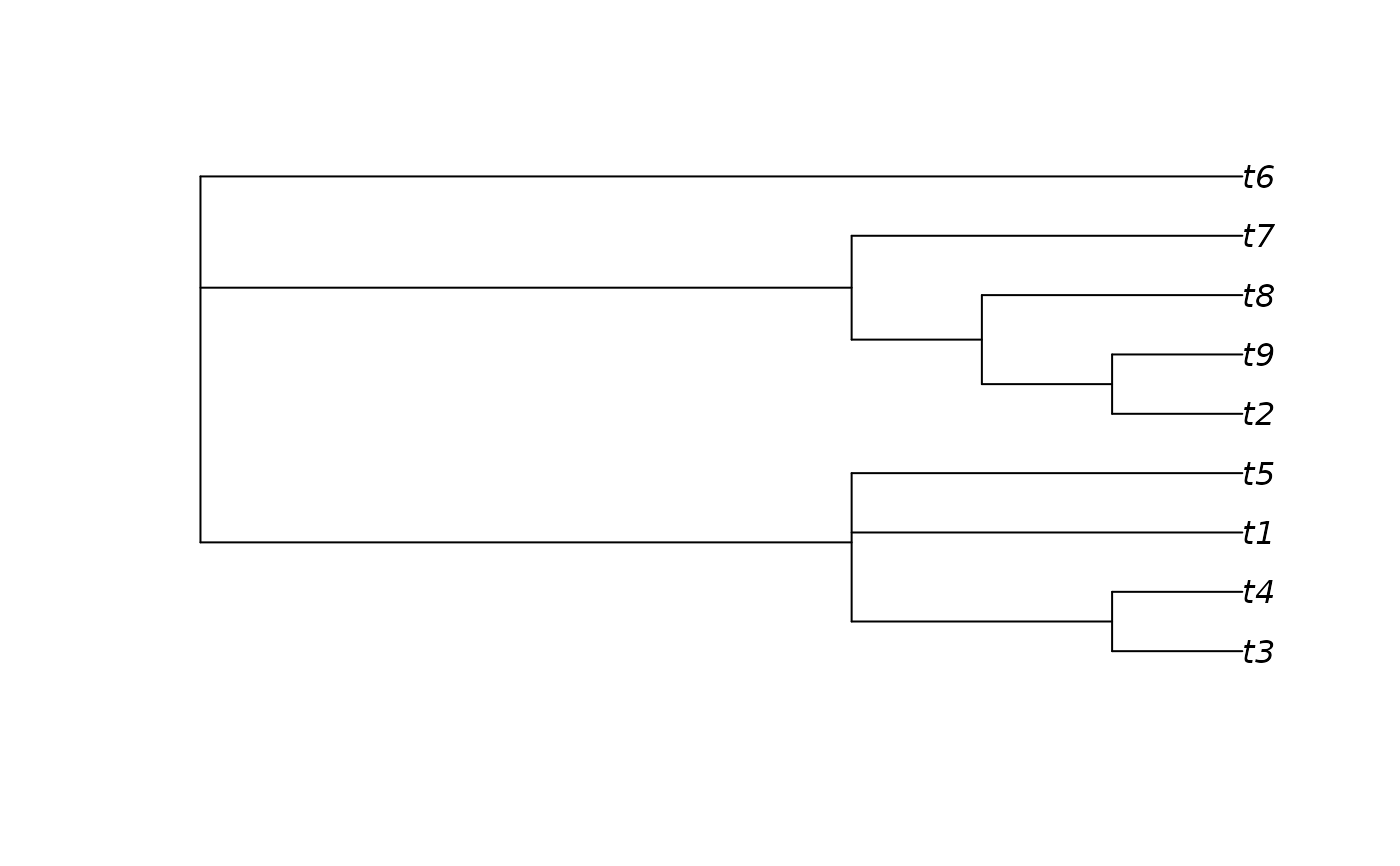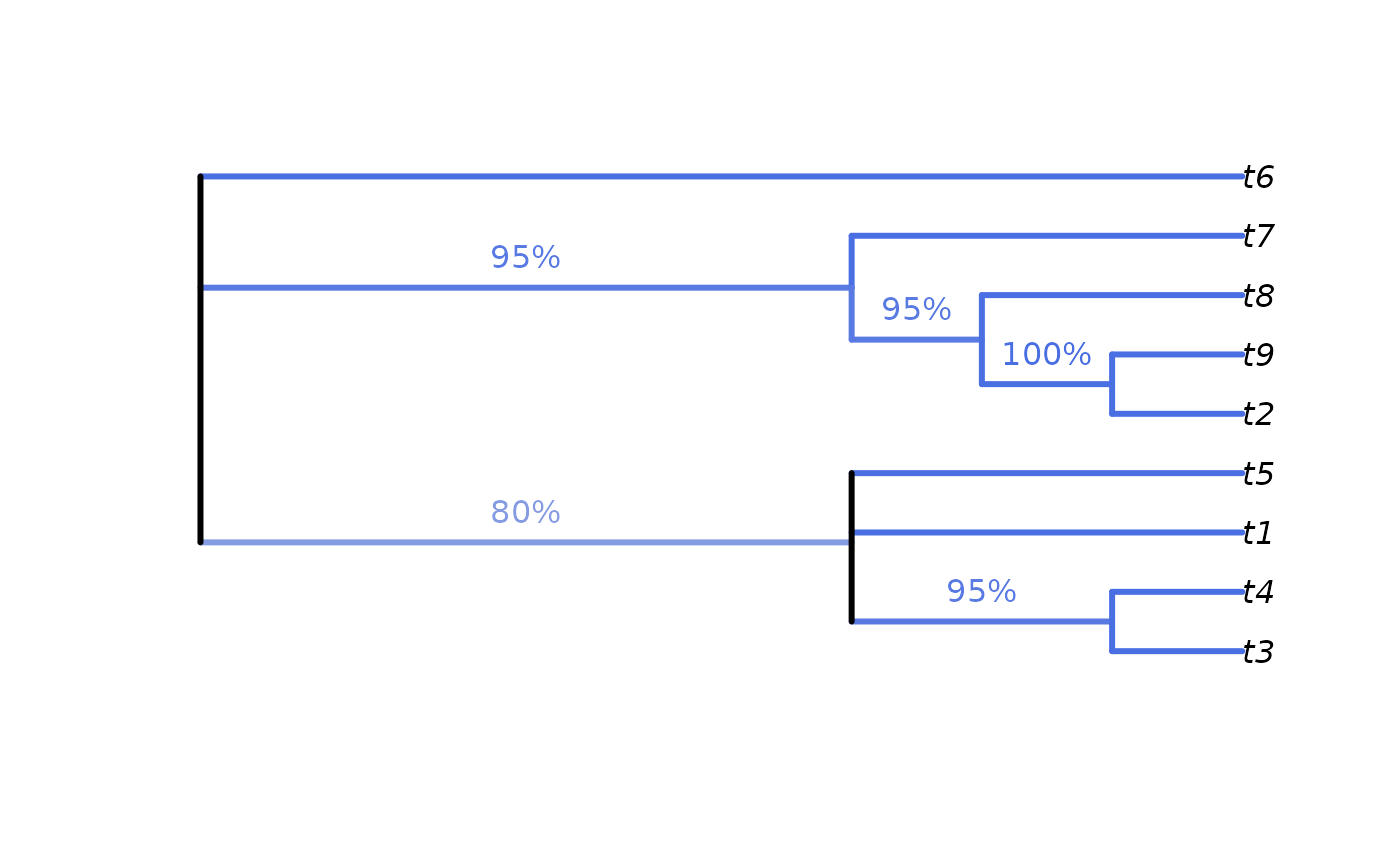Colour value with which to display node support.

SupportColour(
support,
show1 = TRUE,
scale = rev(diverge_hcl(101, h = c(260, 0), c = 100, l = c(50, 90), power = 1)),
outOfRange = "red"
)

SupportColor(
support,
show1 = TRUE,
scale = rev(diverge_hcl(101, h = c(260, 0), c = 100, l = c(50, 90), power = 1)),
outOfRange = "red"
)

## Arguments

support

A numeric vector of values in the range 0--1.

show1

Logical specifying whether to display values of 1. A transparent white will be returned if FALSE.

scale

101-element vector listing colours in sequence. Defaults to a diverging HCL scale.

outOfRange

Colour to use if results are outside the range 0--1.

## Value

SupportColour() returns the appropriate value from scale, or outOfRange if a value is outwith the valid range.

Use in conjunction with LabelSplits() to colour split labels, possibly calculated using SplitFrequency().

## Examples

SupportColour((-1):4 / 4, show1 = FALSE)
#>  "red"       "#D33F6A"   "#E495A5"   "#E2E2E2"   "#9DA8E2"   "#ffffff00"

# An example forest of 100 trees, some identical
forest <- as.phylo(c(1, rep(10, 79), rep(100, 15), rep(1000, 5)), nTip = 9)

# Generate an 80% consensus tree
cons <- ape::consensus(forest, p = 0.8)
plot(cons)# Calculate split frequencies
splitFreqs <- SplitFrequency(cons, forest)

# Optionally, colour edges by corresponding frequency.
# Note that not all edges are associated with a unique split
# (and two root edges may be associated with one split - not handled here)
edgeSupport <- rep(1, nrow(cons$edge)) # Initialize trivial splits to 1 childNode <- cons$edge[, 2]
edgeSupport[match(names(splitFreqs), childNode)] <- splitFreqs / 100

plot(cons, edge.col = SupportColour(edgeSupport), edge.width = 3)

# Annotate nodes by frequency
LabelSplits(cons, splitFreqs, unit = "%",
col = SupportColor(splitFreqs / 100),
frame = "none", pos = 3L)